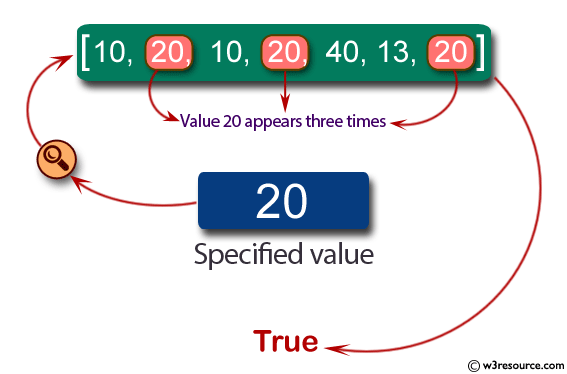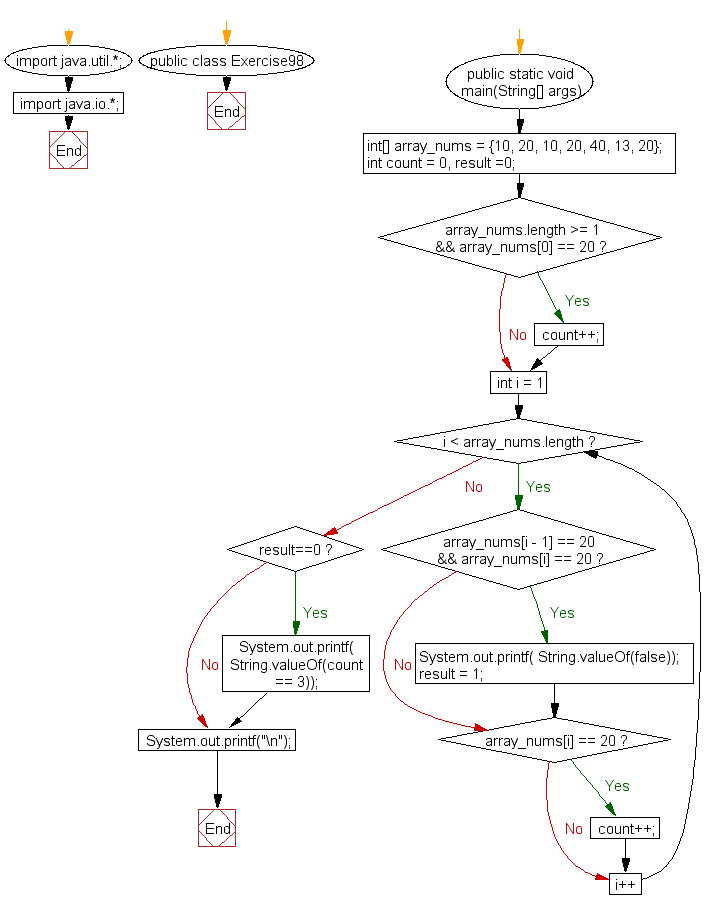﻿ Java exercises: Check if the value 20 appears three times and no 20's are next to each other in a given array of integers - w3resource# Java Exercises: Check if the value 20 appears three times and no 20's are next to each other in a given array of integers

## Java Basic: Exercise-98 with Solution

Write a Java program to check if the value 20 appears three times and no 20’s are next to each other in a given array of integers.

Pictorial Presentation:Sample Solution:

Java Code:

``````import java.util.*;
import java.io.*;
public class Exercise98 {
public static void main(String[] args)
{
int[] array_nums = {10, 20, 10, 20, 40, 13, 20};

int count = 0, result =0;

if(array_nums.length >= 1 && array_nums == 20)
count++;

for(int i = 1; i < array_nums.length; i++) {
if(array_nums[i - 1] == 20 && array_nums[i] == 20)
{
System.out.printf( String.valueOf(false));
result = 1;
}
if(array_nums[i] == 20)
count++;
}

if (result==0)
{
System.out.printf( String.valueOf(count == 3));
}
System.out.printf("\n");
}
}
```
```

Sample Output:

```true
```

Flowchart:Java Code Editor:

What is the difficulty level of this exercise?

﻿

## Java: Tips of the Day

Execution of console commands:

Java allows you to execute console commands directly from code using a class Runtime. It is very important not to forget about exception handling.

For example, let's try to open a PDF file through a Java terminal:

```import java.io.BufferedReader;
import java.io.InputStream;

public class ShellCommandExec {

public static void main(String[] args) {
String gnomeOpenCommand = "gnome-open //home//user//Documents//MyDoc.pdf";

try {
Runtime rt = Runtime.getRuntime();
Process processObj = rt.exec(gnomeOpenCommand);

InputStream stdin = processObj.getErrorStream();

String myoutput = "";